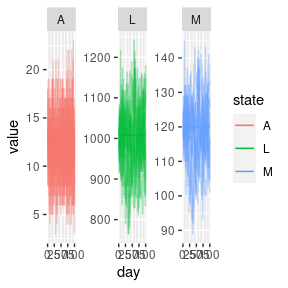# Beverton-Holt aquatic model

library(MicroMoB)
library(ggplot2)
library(data.table)
library(parallel)

The simplest non-trivial model of aquatic mosquito dynamics which exhibits density-dependence (negative feedback) is a Beverton-Holt (BH) style model. It is a kind of discrete logistic equation. We have implemented a BH model that conforms to the aquatic (immature) component interface to be used with other mosquito models fulfilling the adult component interface.

The model groups all immature mosquito stages into a single compartment L. Each time step some number may mature and be ready to emerge as adults, A. The model requires 3 (potentially time and patch varying parameters), K, which determines the strength of density dependent mortality, surv, the baseline daily survival probability, and molt, the daily proportion of immature mosquitoes which mature and emerge as adults.

## Deterministic model

The state updating equation is as follows:

$\begin{equation} L_{t+1} = eggs + (1-molt) \cdot surv \cdot L_{t} \cdot \left( \frac{K}{L_{t}+K} \right) \\ A_{t} = molt \cdot surv \cdot L_{t} \cdot \left( \frac{K}{L_{t}+K} \right) \end{equation}$

## Stochastic model

In the stochastic model on a time step, we first draw the number of survivors:

$\begin{equation} survivors \sim \mathrm{binomial}(L, surv \cdot \left( \frac{K}{L_{t}+K} \right) ) \end{equation}$

The number who emerge is also a binomial random variate:

$\begin{equation} emerge \sim \mathrm{binomial}(survivors, molt) \end{equation}$

Then state is updated:

$\begin{equation} L_{t+1} = eggs + survivors - emerge \\ A_{t} = emerge \end{equation}$

## Deterministic equilibrium

Despite being a nonlinear equation, we can solve the equilibrium. We assume that eggs is known, and that the desired daily emergence is known (lambda, or A in the BH model’s notation). Then we solve for K and L:

$\begin{equation} L = \lambda \left( \frac{1}{molt} - 1 \right) + eggs \\ K = \frac{-\lambda L}{\lambda - L \cdot molt \cdot surv} \end{equation}$

We can set up a simulation using the RM mosquito model to check that everything is at equilibrium. First we calculate the equilibrium values. We do not use any components other than the aquatic and adult mosquito components.

patches <- 1
tmax <- 1e2

M <- 120
p <- 0.9
lambda <- M*(1-p)

nu <- 25
f <- 0.3
eggs <- nu * f * M

# static pars
molt <-  0.1
surv <- 0.9

# solve L
L <- lambda * ((1/molt) - 1) + eggs
K <- - (lambda * L) / (lambda - L*molt*surv)

Let’s set up the model. We use make_MicroMoB() to set up the base model object, and setup_aqua_BH() for the Beverton-Holt aquatic model with our chosen parameters. setup_mosquito_RM() will set up a Ross-Macdonald model of adult mosquito dynamics.

We run a deterministic simulation and store output in a matrix.

# deterministic run
mod <- make_MicroMoB(tmax = tmax, p = patches)
setup_aqua_BH(model = mod, stochastic = FALSE, molt = molt, surv = surv, K = K, L = L)
setup_mosquito_RM(model = mod, stochastic = FALSE, f = f, q = 0.9, eip = 10, p = p, psi = diag(1), nu = nu, M = M, Y = 0, Z = 0)

out_det <- data.table::CJ(day = 1:tmax, state = c('L', 'A', 'M'), value = NaN)
out_det <- out_det[c('L', 'A', 'M'), on="state"]
data.table::setkey(out_det, day)

while (get_tnow(mod) <= tmax) {

step_aqua(model = mod)
step_mosquitoes(model = mod)

out_det[day == get_tnow(mod) & state == 'L', value := mod$aqua$L]
out_det[day == get_tnow(mod) & state == 'A', value := mod$aqua$A]
out_det[day == get_tnow(mod) & state == 'M', value := mod$mosquito$M]

mod$global$tnow <- mod$global$tnow + 1L
}

Now we run the same model, but using the option stochastic = TRUE for our dynamics, and draw 10 trajectories.

# stochastic runs
out_sto <- mclapply(X = 1:10, FUN = function(runid) {

mod <- make_MicroMoB(tmax = tmax, p = patches)
setup_aqua_BH(model = mod, stochastic = TRUE, molt = molt, surv = surv, K = K, L = L)
setup_mosquito_RM(model = mod, stochastic = TRUE, f = f, q = 0.9, eip = 10, p = p, psi = diag(1), nu = nu, M = M, Y = 0, Z = 0)

out <- data.table::CJ(day = 1:tmax, state = c('L', 'A', 'M'), value = NaN)
out <- out[c('L', 'A', 'M'), on="state"]
data.table::setkey(out, day)

while (get_tnow(mod) <= tmax) {

step_aqua(model = mod)
step_mosquitoes(model = mod)

out[day == get_tnow(mod) & state == 'L', value := mod$aqua$L]
out[day == get_tnow(mod) & state == 'A', value := mod$aqua$A]
out[day == get_tnow(mod) & state == 'M', value := mod$mosquito$M]

mod$global$tnow <- mod$global$tnow + 1L
}

out[, 'run' := as.integer(runid)]
return(out)
})

out_sto <- data.table::rbindlist(out_sto)

Now we process the output and plot the results. Deterministic solutions are solid lines and each stochastic trajectory is a faint line.

ggplot(out_sto) +
geom_line(aes(x = day, y = value, color = state, group = run), alpha = 0.35) +
geom_line(data = out_det, aes(x = day, y = value, color = state)) +
facet_wrap(. ~ state, scales = "free")